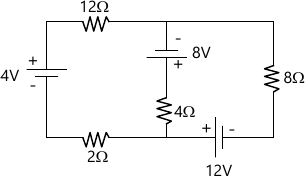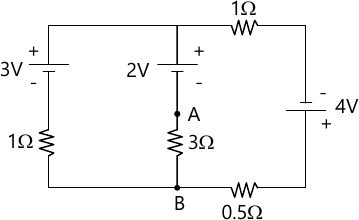MORE IN Basic Electrical Engineering (BEE)
SPPU First Year Engineering (Semester 1)
Basic Electrical Engineering (BEE)
May 2014
Total marks: --
Total time: --
INSTRUCTIONS
(1) Assume appropriate data and state your reasons
(2) Marks are given to the right of every question
(3) Draw neat diagrams wherever necessary

Answer any one question from Q1 and Q2
1 (a) With usual notations: derive the relationship, $\alpha_2 = \dfrac {a_1}{1+\alpha_1 (t_2-t_1)}$ And hence obtain $i) \ \ a_t = \dfrac{a_0}{1+a_0t} \\ i) \ \ a_0 = \dfrac {a_t}{1-a_t t}$
6 M
1 (b) A coil of N turns is wound on a cast iron ring which has mean length of 50 cm and its cross-section is of 4 cm diameter. The current flowing through the coil is 2 A which produces a flux Of 6 nWb in the air-gap of 2mm length. If the relative permeability of iron is 1000, calculate the number of turns N.
6 M

2 (a) With reference to a magnetic circuit explain the teRMS-i) magnetic flux, ii) magneto-motive force, iii) magnetic field intensity, iv) magnetic flux density, v) reluctance, vi) permeability of free space. State their units.
6 M
2 (b) A pump driven by a DC electric motor lifts 1.5 m3 of water per minute to a height of 40 m. The pump has an efficiency of 90 % and the motor an efficiency of 85%. Determine (i) the power input to the motor, (ii) the current taken from a 480 V supply. Assume the mass of one m3 of water is 1000 kg.
6 M

Answer any one question from Q3 and Q4
3 (a) Draw neat sketches to show core-type and shell-type single phase transformers. State:
i) losses occurring in the transformer on load.
ii) their location, cause, whether constant or variable.
iii) factors on which they depends
6 M
3 (b) A series of combination having R= 2MΩ and C= 0.01 μF is connected across the DC source of 50 V. Determine the capacitor voltage and charging current after 0.02s and 0.06s.
6 M

4 (a) Derive an expression for instantaneous current and power consumed when voltage of V= Vm Sin (wt) is applied to pure inductance alone. Also draw the phasor diagram.
6 M
4 (b) A transformer is rated at 100 KVA. At full load, its copper loss is 1200 W and its iron loss is 960 W. Calculate:
(i) Efficiency at full load, 0.8 power factor,
ii) Load KVA at which maximum efficiency will occur.
(iii) Maximum efficiency at 0.85 power factor
6 M

Answer any one question from Q5 and Q6
5 (a) Three identical coils, each having resistance of 15Ω and inductance of 0.03 H, are connected in delta across a 3 phase ,400 V,50 Hz supply. Calculate:
(i) the phase current,
(ii) the line current,
(iii) the power consumed
7 M
5 (b) Derive the expression for line voltage in teRMS of phase Voltage for three phase star connected balanced load, with phasor diagram across three phase supply.
6 M

6 (a) An emf given by v=100 sin 100πt is impressed across a circuit consisting of resistance of 40π in series with 100 μF capacitor and 0.25 H inductor. Determine:(i) RMS value of the current, (ii) power consumed, (iii) power factor.
7 M
6 (b) For a single phase A.C. circuit, the applied voltage is v=Vmsin ωt and current drawn is i=Im sin (ωt-ϕ). Derive expression for average power. Draw waveform of voltage, current and instantaneous power over one cycle of voltage.
6 M

Answer any one question from Q7 and Q8
7 (a) State and explain Thevenin's Theorem.
6 M
7 (b) Apply Kirchhoff's Law to calculate current drawn by 4Ω resistance for the circuit.7 M

8 (a) Derive formula to convert DELTA connected network into its STAR connected equivalent circuit.
6 M
8 (b) Apply superposition theorem to calculate current flowing in 3Ω resistance for the network.7 M

More question papers from Basic Electrical Engineering (BEE)